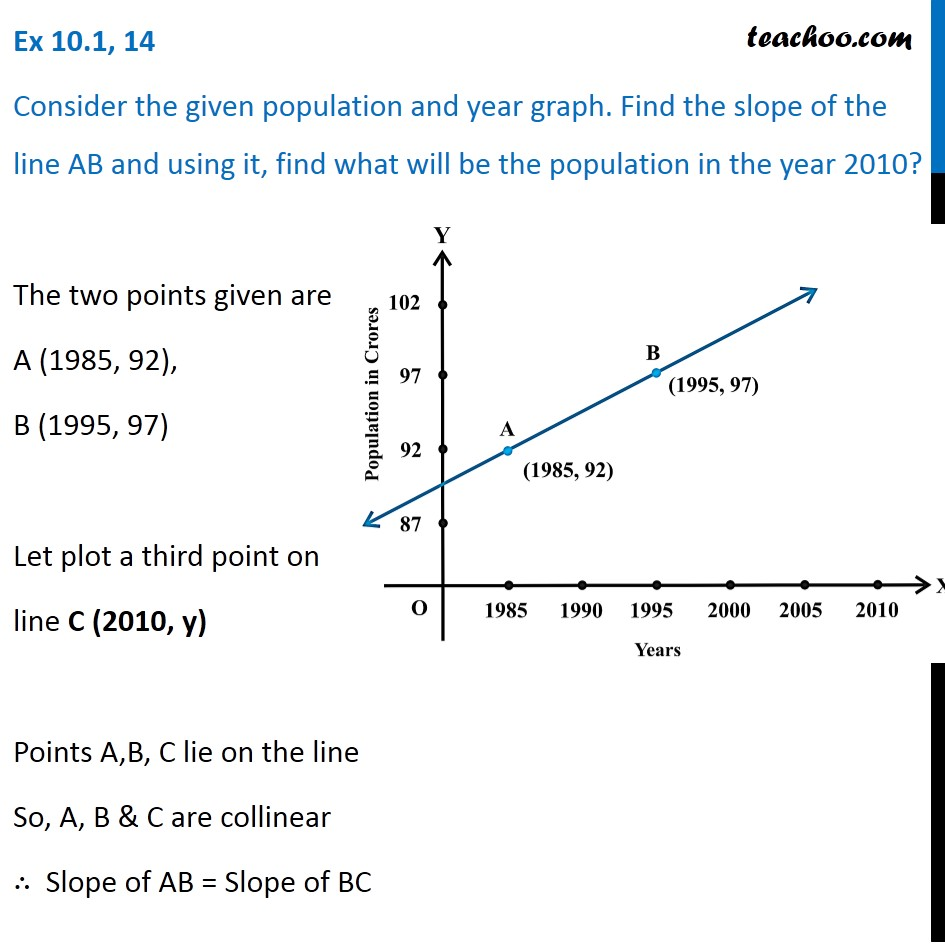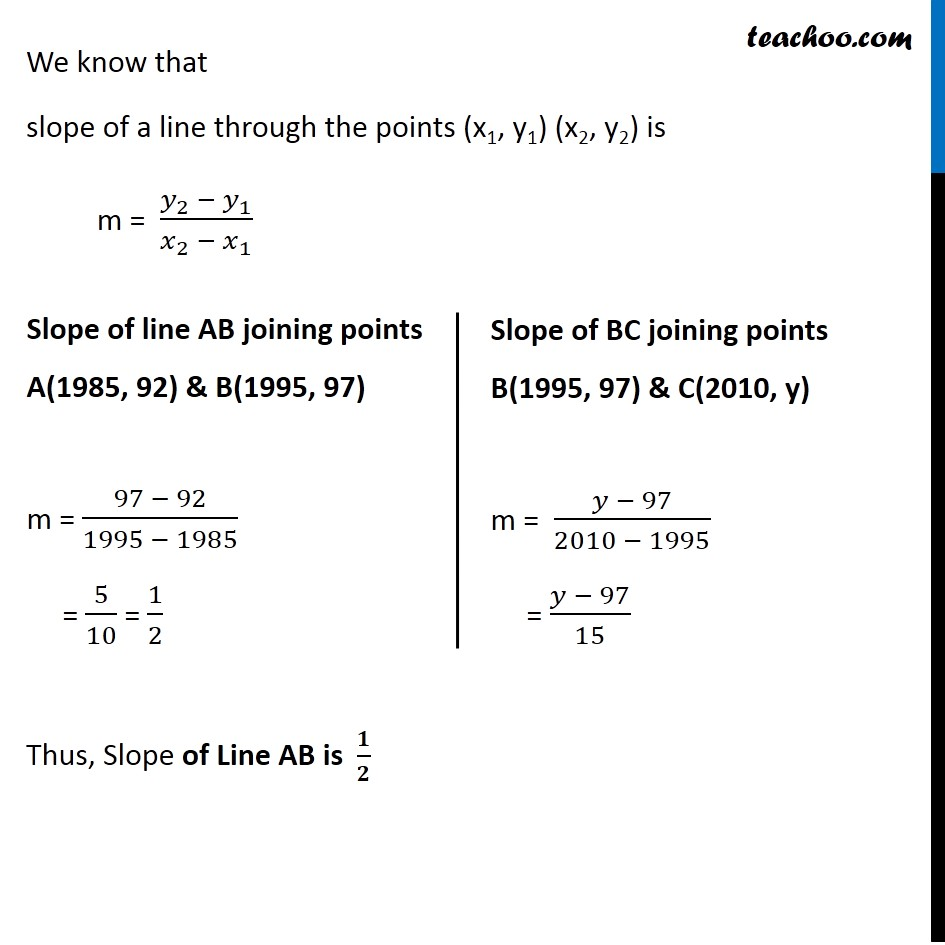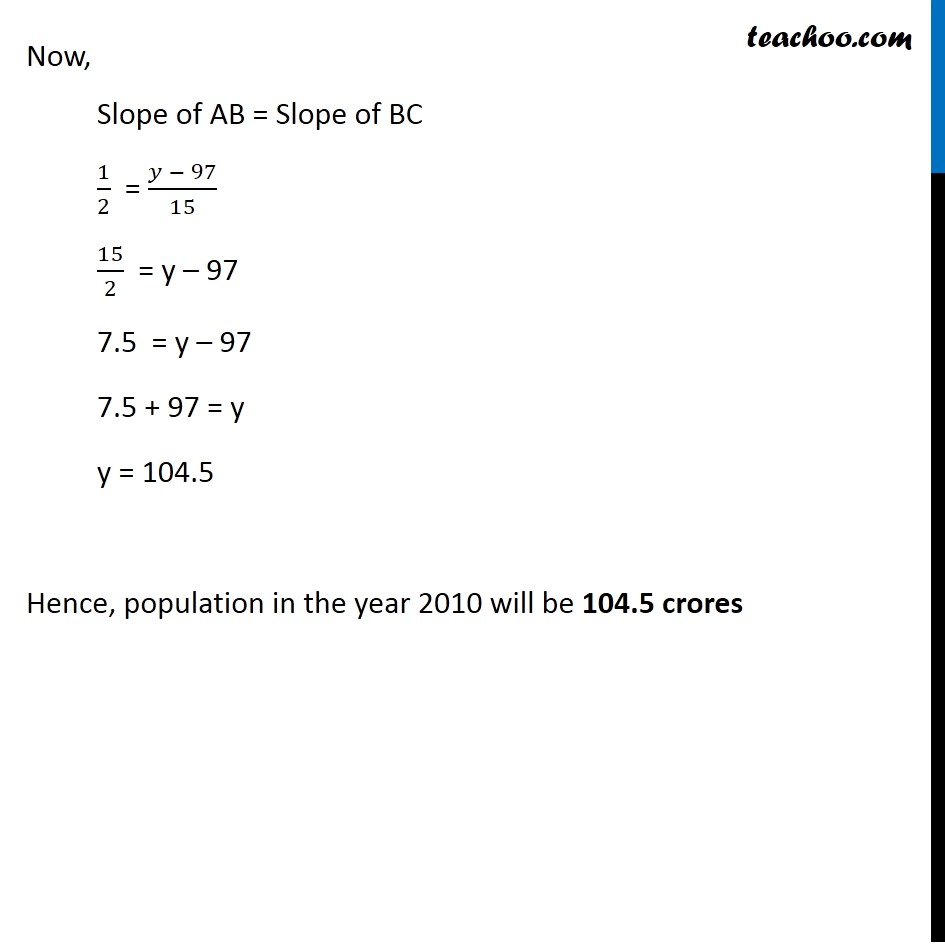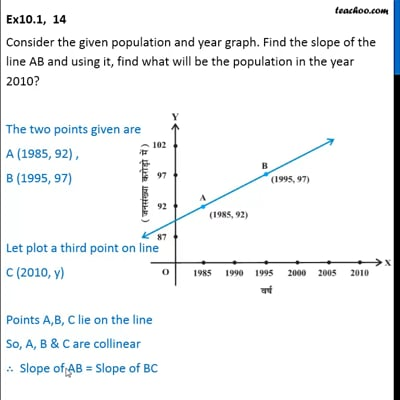Ex 10.1

Chapter 10 Class 11 Straight Lines
Serial order wiseThis video is only available for Teachoo black users

Introducing your new favourite teacher - Teachoo Black, at only ₹83 per month

### Transcript

Ex 10.1, 14 Consider the given population and year graph. Find the slope of the line AB and using it, find what will be the population in the year 2010? The two points given are A (1985, 92), B (1995, 97) Let plot a third point on line C (2010, y) Points A,B, C lie on the line So, A, B & C are collinear ∴ Slope of AB = Slope of BC We know that slope of a line through the points (x1, y1) (x2, y2) is m = (𝑦_2 − 𝑦_1)/(𝑥_2 − 𝑥_1 ) Slope of line AB joining points A(1985, 92) & B(1995, 97) m = (97 − 92)/(1995 − 1985) = 5/10 = 1/2 Slope of BC joining points B(1995, 97) & C(2010, y) m = (𝑦 − 97)/(2010 − 1995) = (𝑦 − 97)/15 Thus, Slope of Line AB is 𝟏/𝟐 Now, Slope of AB = Slope of BC 1/2 = (𝑦 − 97)/15 15/2 = y – 97 7.5 = y – 97 7.5 + 97 = y y = 104.5 Hence, population in the year 2010 will be 104.5 crores